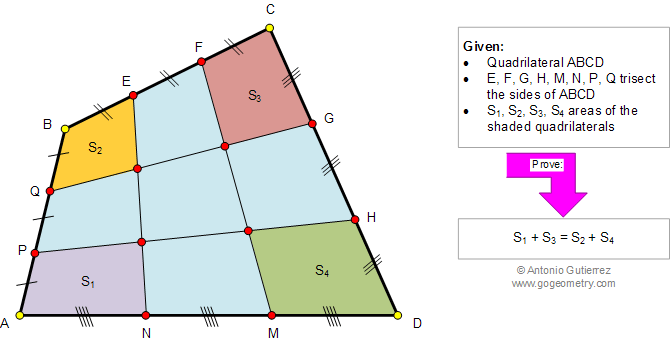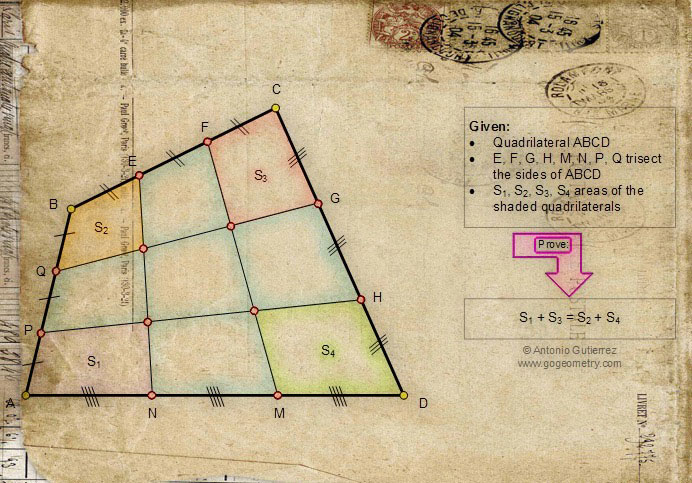# Geometry Problem 961. Quadrilateral, Trisection, Sides, Sum of Areas, School, College, Sketch, Software

< PREVIOUS PROBLEM  |  NEXT PROBLEM >The figure below shows a quadrilateral ABCD. Points E, F, G, H, M, N, P, and Q trisect the sides (divide each side into three equal parts). If S1, S2, S3, and S4 are the areas of the shaded quadrilaterals, prove that: S1 + S3, = S2 + S4.## Sketch of problem 961Problems
All Problems
Open Problems
Visual Index
Ten Problems 961-970